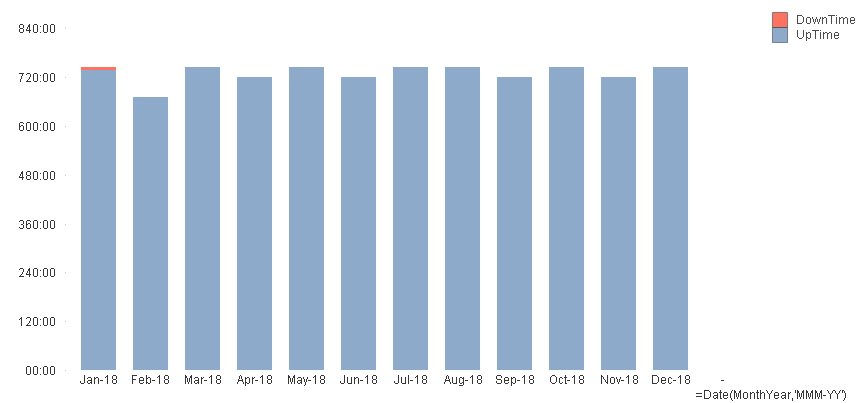# New to QlikView

Discussion board where members can get started with QlikView.

New Contributor III

## Set analysis with variable in bar chart

I have two variables set up:

• vMonthHrs = Interval((Max([SchedDate])-Min([SchedDate])+1) //this gives the number of hours in each month
• vDownTime = Interval(Sum({<QState={'Off'}>}QTime))

I then have a bar chart for the year with a dimension of MonthYear and two expressions:

• Uptime=Interval(\$(vMonthHrs)-\$(vDownTime))
• Downtime=Interval(\$(vDownTime))

which appears to work perfectly if I select all the months.What I need is to just select February and have it still show the bars ... so ignore the Month selection. However when I try to add set analysis ({<Month>} which is what works in all the other charts without variables) I get "No data to display".

I've clearly not quite gotten the hang of set analysis.

Tags (3)
1 Solution

Accepted Solutions
Honored Contributor III

## Re: Set analysis with variable in bar chart

You need to put ({<Month>} in all the aggregation functions, can you share your final expression?

May be like this -

• vMonthHrs = Interval((Max({<Month>}[SchedDate])-Min({<Month>}[SchedDate])+1) //this gives the number of hours in each month
• vDownTime = Interval(Sum({<QState={'Off'},Month>}QTime))
2 Replies
Honored Contributor III

## Re: Set analysis with variable in bar chart

You need to put ({<Month>} in all the aggregation functions, can you share your final expression?

May be like this -

• vMonthHrs = Interval((Max({<Month>}[SchedDate])-Min({<Month>}[SchedDate])+1) //this gives the number of hours in each month
• vDownTime = Interval(Sum({<QState={'Off'},Month>}QTime))
New Contributor III

## Re: Set analysis with variable in bar chart

Thank you. It hadn't occurred to me to put the set analysis in the variable definition.